# NCERT Solutions for Class 8 Maths Chapter 2 Linear Equations in One Variable Ex 2.3

NCERT Solutions for Class 8 Maths Chapter 2 Linear Equations in One Variable Ex 2.3

### NCERT Solutions for Class 8 Maths Chapter 2 Linear Equations in One Variable Exercise 2.3

Solve the following equations and check your results.
Ex 2.3 Class 8 Maths Question 1.
3x = 2x + 18
Solution:
We have 3x = 2x + 18
⇒ 3x – 2x = 18 (Transposing 2x to LHS)
⇒ x = 18
Hence, x = 18 is the required solution.
Check: 3x = 2x + 18
Putting x = 18, we have
LHS = 3 × 18 = 54
RHS = 2 × 18 + 18 = 36 + 18 = 54
LHS = RHS
Hence verified.

Ex 2.3 Class 8 Maths Question 2.
5t – 3 = 3t – 5
Solution:
We have 5t – 3 = 3t – 5
⇒ 5t – 3t – 3 = -5 (Transposing 3t to LHS)
⇒ 2t = -5 + 3 (Transposing -3 to RHS)
⇒ 2t = -2
⇒ t = -2 ÷ 2
⇒ t = -1
Hence t = -1 is the required solution.
Check: 5t – 3 = 3t – 5
Putting t = -1, we have
LHS = 5t – 3 = 5 × (-1)-3 = -5 – 3 = -8
RHS = 3t – 5 = 3 × (-1) – 5 = -3 – 5 = -8
LHS = RHS
Hence verified.

Ex 2.3 Class 8 Maths Question 3.
5x + 9 = 5 + 3x
Solution:
We have 5x + 9 = 5 + 3x
⇒ 5x – 3x + 9 = 5 (Transposing 3x to LHS) => 2x + 9 = 5
⇒ 2x = 5 – 9 (Transposing 9 to RHS)
⇒ 2x = -4
⇒ x = -4 ÷ 2 = -2
Hence x = -2 is the required solution.
Check: 5x + 9 = 5 + 3x
Putting x = -2, we have
LHS = 5 × (-2) + 9 = -10 + 9 = -1
RHS = 5 + 3 × (-2) = 5 – 6 = -1
LHS = RHS
Hence verified.

Ex 2.3 Class 8 Maths Question 4.
4z + 3 = 6 + 2z
Solution:Hence verified.

Ex 2.3 Class 8 Maths Question 5.
2x – 1 = 14 – x
Solution:
We have 2x – 1 = 14 – x
⇒ 2x + x = 14 + 1 (Transposing x to LHS and 1 to RHS)
⇒ 3x = 15
⇒ x = 15 ÷ 3 = 5
Hence x = 5 is the required solution.
Check: 2x – 1 = 14 – x
Putting x = 5
LHS we have 2x – 1 = 2 × 5 – 1 = 10 – 1 = 9
RHS = 14 – x = 14 – 5 = 9
LHS = RHS
Hence verified.

Ex 2.3 Class 8 Maths Question 6.
8x + 4 = 3(x – 1) + 7
Solution:
We have 8x + 4 = 3(x – 1) + 7
⇒ 8x + 4 = 3x – 3 + 7 (Solving the bracket)
⇒ 8x + 4 = 3x + 4
⇒ 8x – 3x = 4 – 4 [Transposing 3x to LHS and 4 to RHS]
⇒ 5x = 0
⇒ x = 0 ÷ 5 [Transposing 5 to RHS]
or x = 0
Thus x = 0 is the required solution.
Check: 8x + 4 = 3(x – 1) + 7
Putting x = 0, we have
8 × 0 + 4 = 3(0 – 1) + 7
⇒ 0 + 4 = -3 + 7
⇒ 4 = 4
LHS = RHS
Hence verified.

Ex 2.3 Class 8 Maths Question 7.Hence verified.

Ex 2.3 Class 8 Maths Question 8.Ex 2.3 Class 8 Maths Question 9.Solution:Ex 2.3 Class 8 Maths Question 10.
3m = 5m – 8/5
Solution:
We have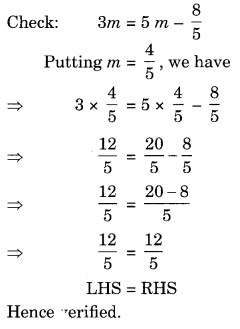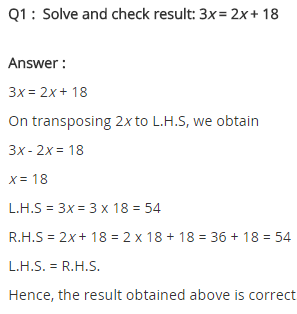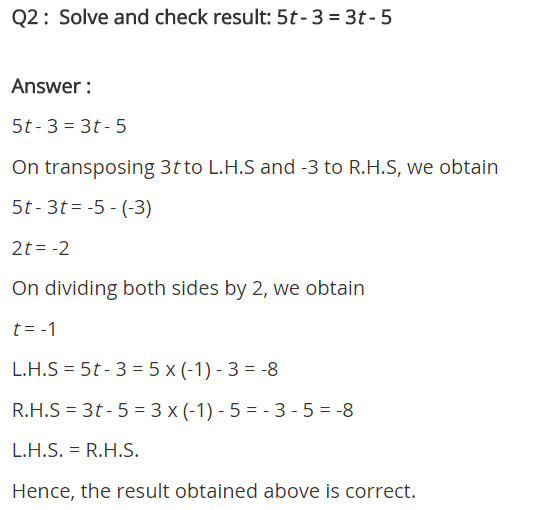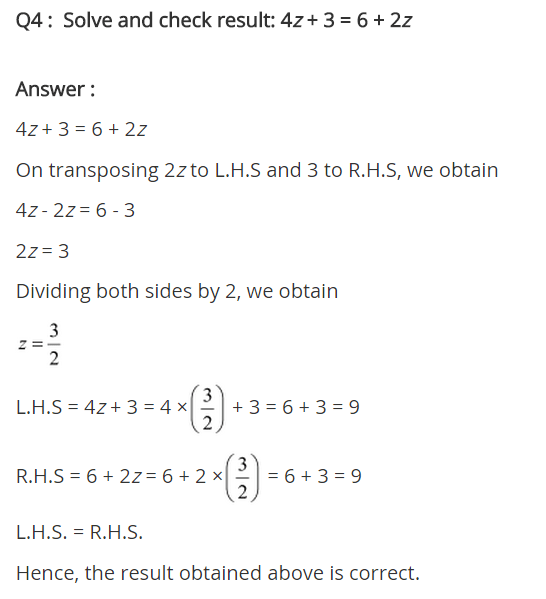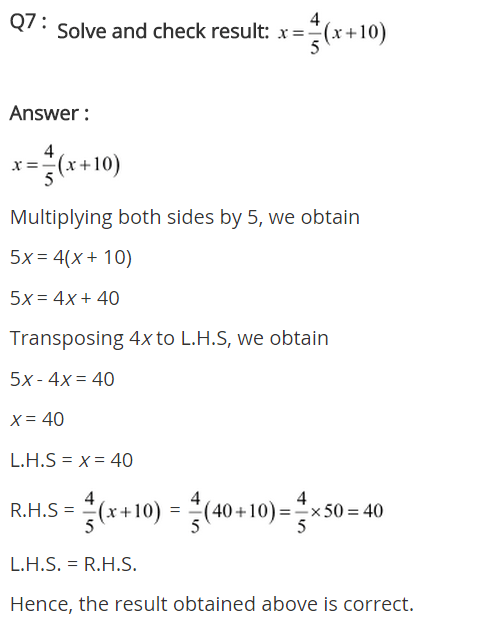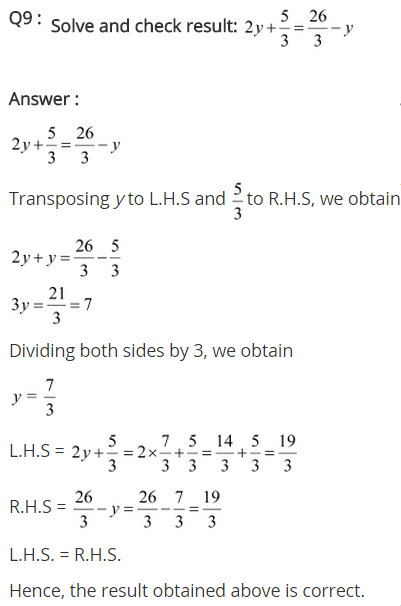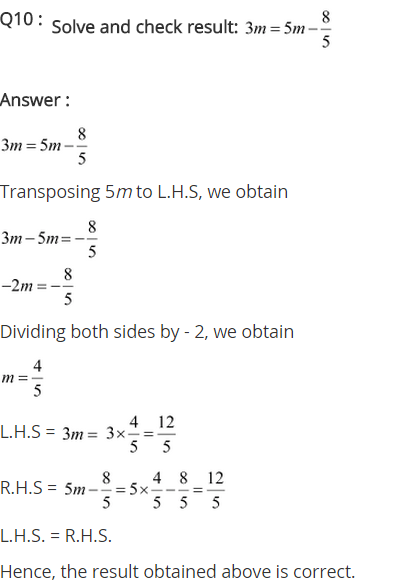## SabDekho

The Complete Educational Website